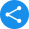# NCERT Solutions for Class 4View Notes

## Class 4 NCERT Solutions for Maths, English, EVS & Hindi

Develop logical and reasoning skills with the proper explanation of concepts in the NCERT solutions Class 4 pdf. These solutions cover the entire CBSE curriculum and focus on fundamentals; thus, helpful for students. After a lot of research, NCERT solutions Class 4 prepared by experts help to build a robust foundation on every subject. These solutions offer thorough and tremendous knowledge about the topic in a simple and easy-to-understand language.

### NCERT Solutions for Class 4

1. What Topics are Covered in class 4 Mathematics?

Ans: Mathematics subject covers basic concepts in Class 4 syllabus like Number System, Addition, Factors Multiples Estimation, Subtraction, Patterns, Division, and Multiplication. The students will be asked to solve various Maths problems regarding these concepts. Apart from it, students will go through Symmetry, Geometrical Shapes, Decimal Numbers, Fractional Numbers, Money, Time, Calendar, Data Handling, and more. Students will learn the different decimal numbers into fractional numbers. The topic of geometrical shapes will help students analyse different types of shapes, like a circle, rhombus, trapezium, and more in mathematics.

2. Mention Important Facts Related to NCERT Solutions for Class 4.

Ans: Students must be aware of the importance of NCERT Solutions for Class 4 so that they can get the advantage of it.

• If students are stuck anywhere while solving certain questions, then they need to refer a certain subject NCERT solutions so that they get the right answer.

• If you refer to NCERT solutions, then put in your mind to know how the solution reached the right answer.

• Try to find out the way the solution has been derived using a particular NCERT chapter.

Share this with your friendsShare